Thermodynamics and Propulsion

# 7.3 A Statistical Definition of Entropy

The list of theis a precise description of the randomness in the system, but the number of quantum states in almost any industrial system is so high this list is not useable. We thus look for a single quantity, which is a function of the, that gives an appropriate measure of the randomness of a system. As shown below, the entropy provides this measure.

There are several attributes that the desired function should have. The first is that the average of the function over all of the microstates should have an extensive behavior. In other words the microscopic description of the entropy of a system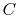, composed of parts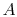and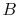should be given by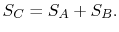(7..4)

Second is that entropy should increase with randomness and should be largest for a given energy when all the quantum states are equiprobable.

The average of the function over all the microstates is defined by(7..5)

where the function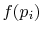is to be found. Suppose that systemhasmicrostates and systemhas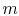microstates. The entropies of systems,, and, are defined by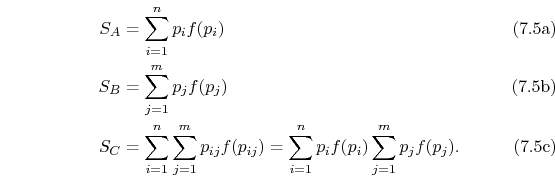In Equations (7.5) and (7.6), the term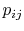means the probability of a microstate in which systemis in stateand systemis in state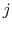. For Equation (7.4) to hold given the expressions in Equations (7.6),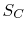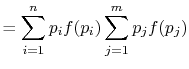(7..7)

The function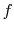must be such that this is true regardless of the values of the probabilitiesand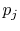. This will occur if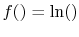because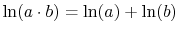.

To verify this, make this substitution in the expression for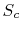in the first part of Equation (7.6c) (assume the probabilitiesandare independent, such that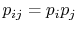, and split the log term):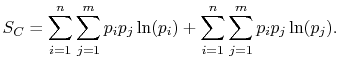(7..8)

Rearranging the sums, (7.8) becomes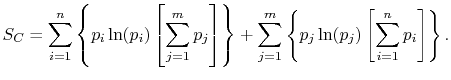(7..9)

Because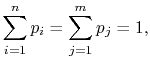(7..10)

the square brackets in the right hand side of Equation (7.9) can be set equal to unity, with the result written as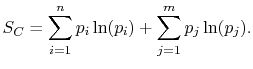(7..11)

This reveals the top line of Equation (7.7) to be the same as the bottom line, for any,,,, provided that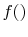is a logarithmic function. Reynolds and Perkins show that the most generalis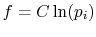, whereis an arbitrary constant. Because theare less than unity, the constant is chosen to be negative to make the entropy positive.

Based on the above, a statistical definition of entropy can be given as:(7..12)

The constantis known as the Boltzmann constant,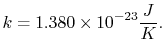(7..13)

The value ofis (another wonderful result!) given by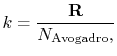(7..14)

where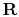is the universal gas constant,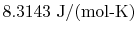and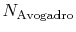is Avogadro's number,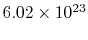molecules per mol. Sometimesis called the gas constant per molecule. With this value for, the statistical definition of entropy is identical with the macroscopic definition of entropy.

UnifiedTP